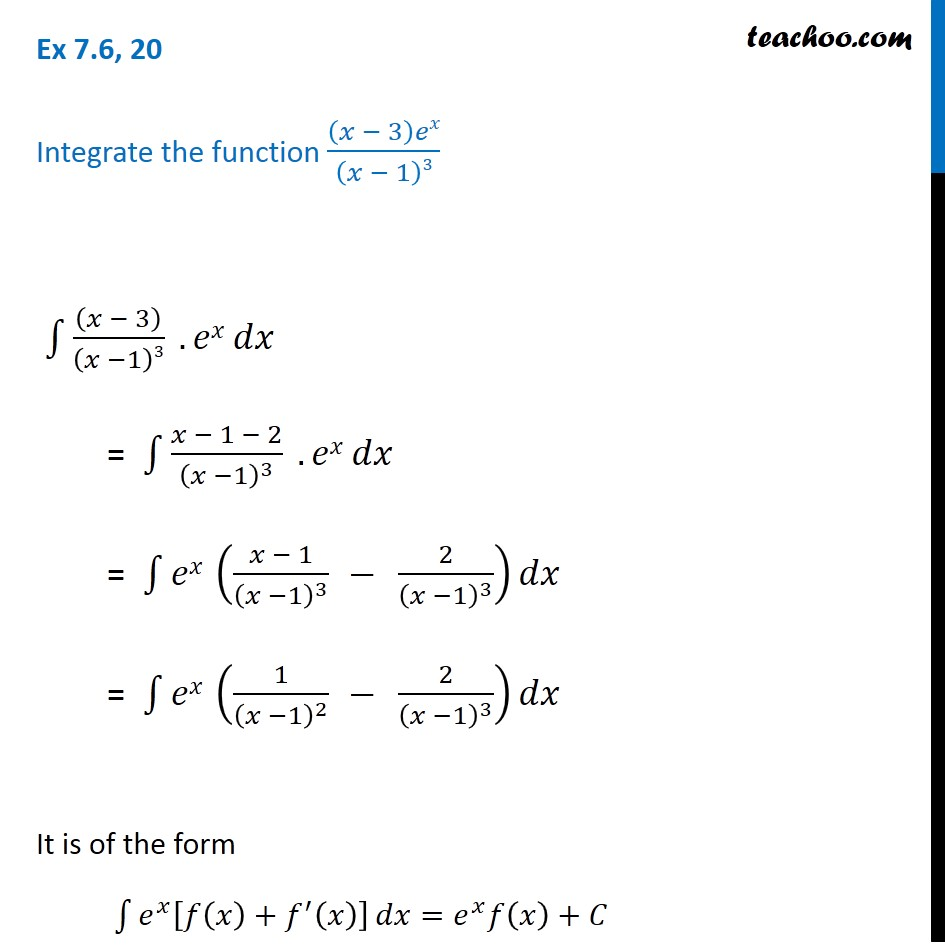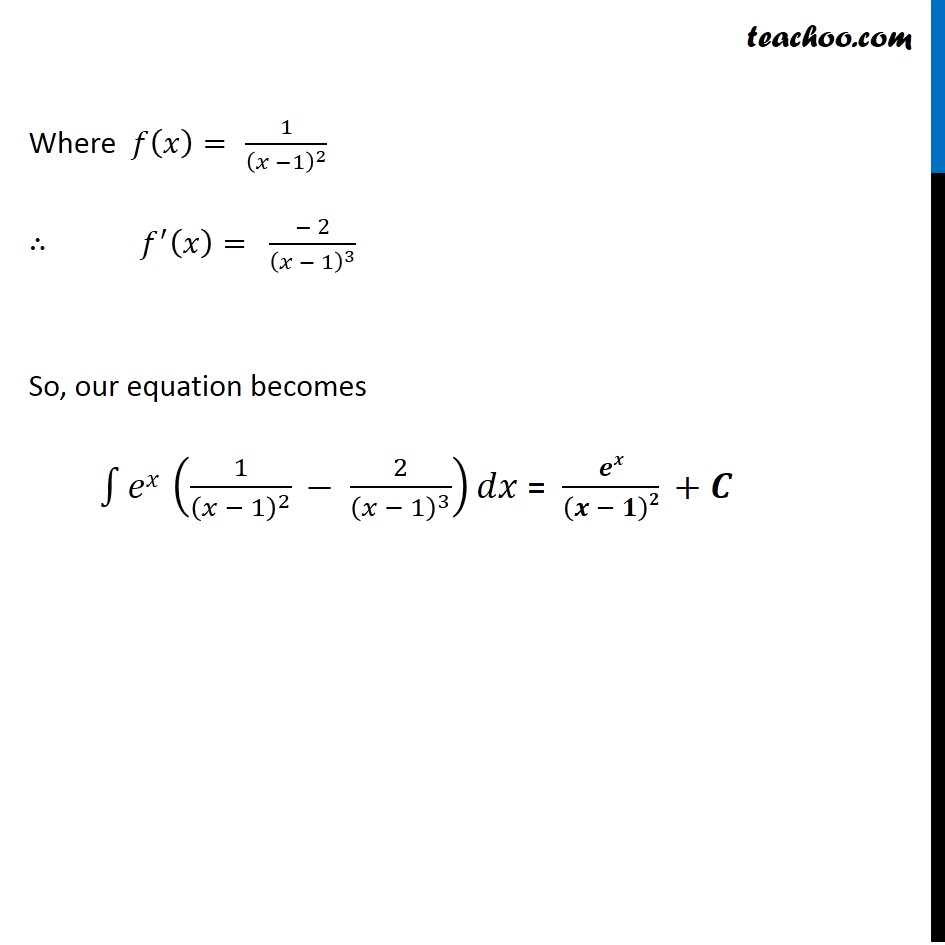1. Chapter 7 Class 12 Integrals (Term 2)
2. Concept wise
3. Integration by parts - e^x integration

Transcript

Ex 7.6, 20 Integrate the function (𝑥 − 3)𝑒𝑥/(𝑥 − 1)3 ∫1▒〖((𝑥 − 3))/(𝑥 −1)3 .𝑒𝑥" " 𝑑𝑥〗 = ∫1▒〖(𝑥 − 1 − 2)/(𝑥 −1)^3 .𝑒𝑥" " 𝑑𝑥〗 = ∫1▒〖𝑒𝑥 ((𝑥 − 1)/(𝑥 −1)^3 − 2/(𝑥 −1)^3 )𝑑𝑥〗 = ∫1▒〖𝑒𝑥 (1/(𝑥 −1)^2 − 2/(𝑥 −1)^3 )𝑑𝑥〗 It is of the form ∫1▒〖𝑒^𝑥 [𝑓(𝑥)+𝑓^′ (𝑥)] 〗 𝑑𝑥=𝑒^𝑥 𝑓(𝑥)+𝐶 Where 𝑓(𝑥)=" " 1/(𝑥 −1)^2 ∴ 𝑓^′ (𝑥)= (− 2)/(𝑥 − 1)^3 So, our equation becomes ∫1▒〖𝑒𝑥 (1/(𝑥 − 1)^2 − 2/(𝑥 − 1)^3 )𝑑𝑥〗 = 𝒆𝒙/(𝒙 − 𝟏)𝟐 +𝑪

Integration by parts - e^x integration

Chapter 7 Class 12 Integrals (Term 2)
Concept wise